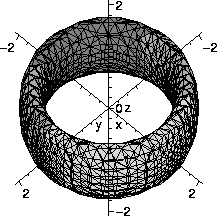Beginning Maple, spring 2006

Only the first recitation meeting (Wednesday, January 18) of sections 5 through 10 of Math 251 will be in an "Instructional Microcomputer Lab" (IML) to get acquainted with Maple. Later recitations will be in your standard scheduled recitation rooms.
• Sections 5, 6, and 7 will meet in IML 119 which is inside the ARC computer lab. Please go there at your regular class times:
• Section 5: 8:40-10:00 AM
• Section 6: 10:20-11:40 AM
• Section 7: 12:00-1:20 PM
• Sections 8, 9, and 10 will meet in IML 118 which is inside the ARC computer lab. Please go there at your regular class times:
• Section 8: 12:00-1:20 PM
• Section 9: 1:40-3:00 PM
• Section 10: 3:20-4:40 PM
Students will work through the following material:

 General introduction 1/6/2006 Playing with arithmetic 1/6/2006 Playing with algebra 1/6/2006 Playing with calculus 1/6/2006 Playing with graphs 1/6/2006

 We've noticed that some printouts of these pages generate weird symbols. The pages as viewed are correct. If your printouts seem strange or difficult to understand, please look at the pages as viewed by your browser.

Why learn Maple?
Almost every aspect of the practice of mathematics, both pure and applied, has been improved and amplified in recent decades by the widespread availability of "computer algebra systems", CAS. This technology is much more than just algebra, of course. It is a collection of systematic and powerful programs that permit algebraic manipulation

• What is the coefficient of x6y4z2 in (x+y+z)12?
13860
and numerical approximation
• What is an approximation to the only root of 3x+cos(2x2)=0?
-.3258460227
and graphing
• What do the points (x,y,z) which satisfy the equation z2+(x2+y2-1)(x2+y2-2)=0 look like?The freedom to work with "exact" symbolic computation, with numerical approximation (with specified accuracy) and with visual display of data (human beings learn much more from pictures than from lists of numbers!) is very useful.

Almost all of my teaching and research is now improved by access to powerful programs which allow me to experiment. I can get examples which are useful for instruction. I've also used these programs to try to understand complicated phenomena which I could not easily explain.

Computer help
Many students have graphing calculators. These are useful, but are limited by speed and memory size. Simple errors may occur. There are large computer programs with powerful numerical, symbolic, and graphical capabilities. These still may have the potential for errors, but much effort has gone into their programming. The most widely distributed programs are Maple, Mathematica, and Derive. In this course Maple will be favored, since almost every large computer system at Rutgers has Maple installed. These programs are not infallible but they can be very helpful. Other programs available with special capabilities. For example, Matlab, a program originally directed at problems of linear algebra, is widely used at the Engineering School.

How to get those answers
The answers to the questions above were obtained with the following Maple instructions. Please: these instructions are not given to impress you, but rather to show how easy is is to get the answers.

• coeff(coeff(coeff((x+y+z)^12,x^6),y^4),z^2);
The command coeff(P,monomial) finds the coefficient of the monomial in the expression P. Layering three repetitions of coeff finds the desired coefficient.

• fsolve(3*x+cos(2*x^2)=0,x);
fsolve is a general "floating point" approximate equation solver. Care must be used if there's more than one root. There are also symbolic solvers, useful when there is a nice formula for the solution.

• with(plots):
V:=((x^2+y^2)-1):
W:=((x^2+y^2)-2):
implicitplot3d(-V*W=z^2,x=-2..2,y=-2..2,z=-2..2,grid=[30,30,30],axes=normal);

The implicitplot3d command sketches graphs which are defined implicitly by equations. Since Maple has so many functions and libraries available, many need to be specifically loaded before use. The command with(plots); loads a variety of plotting commands. The implicitplot3d command has a wide variety of options. The grid option gives control over the spacing of sample points. Of course increasing the number of sample points "costs" computational time.

Programming in Maple
Maple is also a programming environment. Maple programs are called procedures. The Maple language has many statements supporting program flow such as if ...then and while and do etc., and also has a variety of data types. There's no time in this course to teach this material, but students should know that programmng is possible.

I have several books on Maple programming. My current favorite is Maple A comprehensive introduction by Roy Nicolaides and Noel Walkington, Cambridge University Press (\$65, 484 pages, available for less in places on the web). There are also many web pages which discuss programming in Maple. For example, here's one online tutorial. Warning: such pages are only for the enthusiast!

Maintained by greenfie@math.rutgers.edu and last modified 1/6/2006.